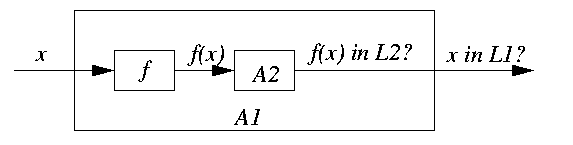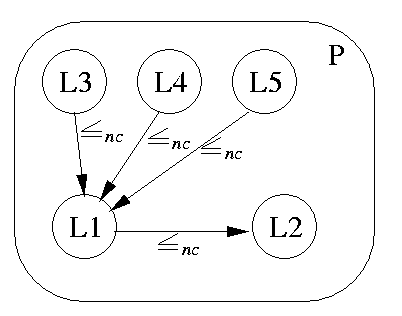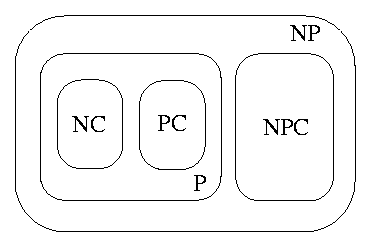## The contents

We have seen that NC is subset of P, but similarly to the NP-completeness theory, the problem whether P=NC is open and is likely equally difficult as its famous predecessor P=NP. The situation is very similar and also the techniques to deal with the problem are similar. Let us demonstrate these similarities in more detail.

Suppose many researchers have worked hard trying to find a highly parallel algorithm for solving some polynomial problem K and suppose they all have failed. This leads to a general, even though unproven, consensus that K is sequential in its essence. Suppose there is another polynomial problem K' which we want to parallelize, but after certain effort we give up, since no highly parallel solution is in sight and we rather start to suspect K' that it is also inherently sequential. Unfortunately, the small amount of work invested into the parallelization of K' is rather poor evidence that K' is really unparallelizable. But suppose that we manage to show that K can be reduced to K' (i.e., K can be described as an algorithm which makes calls to K') and this reduction itself is a highly parallel algorithm in the sense explained in Section 3, i.e., NC. This will support our hypothesis that K' is likely to be inherently sequential, since otherwise, K would become a problem with highly parallel feasibility, which would contradict the current overwhelming experience with K. However, there is still a small chance that K (and consequently K') does have a highly parallel solution, it is just bad luck that it has not been found yet. A much more convincing argument that K' is inherently sequential would be to show that any known hardly parallelizable polynomial problem can be reduced to K' in a highly parallel way. This is exactly the idea of P-completeness.
 Back to the beginning of the page Back to the CS838 class schedule

## Highly parallel reduction

Let us start with an informal example. Consider the following 4 problems:
• Maximum Flow Value Problem (MFV): (functional optimization problem)
Input: Graph G, whose each edge e is labeled by capacity c(e)>= 0, and two vertices s,t.
Problem: Compute the maximum flow f(s,t) from source s to sink t in G.
• Maximum Flow Bit Problem (MFB(i)): (decision problem)
Input: Graph G labeled by capacities, vertices s,t, and integer i.
Problem: Is the i-th bit of the maximum flow f(s,t) equal to 1?
• Maximum Flow Threshold Problem (MFT(k)): (decision problem)
Input: Graph G labeled by capacities, vertices s,t, and integer k.
Problem: Is the maximum flow f(s,t) less than k?
• Maximum Flow Pattern Problem (MFP): (relational optimization problem)
Input: Graph G, edges labeled by capacities, and vertices s,t.
Problem: Compute a flow pattern in G achieving the maximum flow f(s,t).
Parallel reductions allow to compare these problems from the viewpoint of highly parallel feasibility.
• MFB(i) to MFV: trivial and highly parallel: solve MFV and look at the i-th bit.
• MFV to MFB(i): highly parallel:
1. Compute an upper bound M on the value of f(s,t), for example, by summing the capacities of all edges incident to s and t. This is a highly parallel computation. Let m=log M.
2. Solve m instances of MFB(i), i=1,...,m, in parallel.
3. Combine the outputs of MFB(i) into an integer value. Also can be done in parallel.
It follows that MFB(i) is highly parallel iff MFV is highly parallel. This approach can be generalized.

#### Lemma

Computation of any function g:{0,1}*->{0,1}* induces associated bitwise decision problems gi: ``Is bit i of g(x) equal to 1?'' If g(x) is bounded, both problems are of the same parallel complexity.

However, MFT(k) and MFV likely differ in parallel complexity.

• MFT(k) to MFV: trivial and highly parallel: solve MFV and compare the result with k.
• MFV to MFT(i): cannot be likely done in a highly parallel way. Assume that f(s,t) is again bounded by M and let m=log M. We need a sequential binary search performed as a sequence of recursive calls.
• Call MFT(2^{m-1}). If MFT(2^{m-1}) answers NO,
then m-th bit is 1 and call recursively MFT(2^{m-1}+2^{m-2})
else m-th bit is 0 and call recursively MFT(2^{m-2}).
This works fine sequentially, but these m calls cannot be done in parallel. No matter how MFT(k) is feasible in parallel and no matter how many processors we have, we must perform all m calls sequentially, since each of them depends on the result of the preceding one. Consequently, even if MFT(k) had a highly parallel solution, MFV (and therefore MFB(i) as well) reduction on MFT(k) is inherently sequential. This could be an indication that MFT(k) itself is inherently sequential.

Consider now the MFP problem. It is not a functional problem, there may be many flow patterns as solutions. Successive calls to MFP may give different results. Therefore, any parallel reduction of a problem to MFP might give different answers (compare with the reduction of MFV to MFB(i) above). A usual approach is to require some additional unique property, such as being the lexicographically least solution, which converts the problem into a functional one.

Let us now generalize the notion of reducibility. It should not come as a surprise that the highly parallel reduction used intuitively in the example above is called NC-reduction.

#### Definition

A language L1 in P is NC-reducible to a language L2 in P, L1<=nc L2, if there is a function f:{0,1}*->{0,1}* such that for all x in {0,1}* (x in L1 iff f(x) in L2) and f can be computed by an NC algorithm.

Hence, if we are able to show that L1<=nc L2, we have shown that

1. either if L2 in NC, then L1 in NC,
2. or if L1\not in NC, then L2\not in NC.
Therefore, we can
1. either prove an NC upper bound for L2 which gives an NC upper bound for L1,
2. or prove a polynomial lower bound for L1 which gives a polynomial lower bound for L2.
Of course, if L1<=nc L2 and L2<=nc L1, then L1 and L2 are of the same parallel feasibility. And this is a basis for defining the class of P-complete languages/problems.
 Back to the beginning of the page Back to the CS838 class schedule

## P-completeness

#### Definition

A language L is P-hard under NC-reducibility if L'<=nc L for every L' in P.

Informally, a P-hard language L is as hard to decide in parallel as any problem in P.

#### Definition

A language L is P-complete if it is in P and it is P-hard.

Hence, L is in fact in P and all other problems have at most that parallel complexity as L. Similarly, we can define

#### Definition

A function optimization problem K is FP-complete if it is in FP and it is P-hard.

As in the sequential complexity theory, we have two basic observations.#### Lemma

The class NC is closed under the relation of NC-reduction: If L1<=nc L2 and L2 in NC, then L1 in NC.#### Lemma

The class of P-complete problems is closed under the relation of NC-reduction: If L2 in P and there exists P-complete L1 such that L1<=nc L2, then L2 is P-complete.

Corollary:
If any P-complete problem is in NC, then NC=P. If any P-complete problem is provably not to have an NC algorithm, then no P-complete problem is in NC. There is a strong evidence that the second case is true, even though no proof was given up to now. There is a striking similarity between the structure of NP from the viewpoint of sequential feasibility and the structure of P from the viewpoint of highly parallel feasibility.To show that L is P-complete, we have to show that L is in P, and then we have again two choices.

• We have to show that any language in P can be NC-reduced to L. This work had to be done for the first P-complete problems.
• We can select a suitable known P-complete L' and must
1. describe an algorithm computing a function f mapping instances of L' into instances of L,
2. prove that x in L' iff f(x) in L for all x in {0,1}*,
3. prove that f can be computed by an NC algorithm.
The reason behind P-completeness of a problem is usually an unavoidable data dependency within the problem solution, so that the processors cannot work on many parts of the problem simultaneously, and that is why these problems are called inherently sequential.
 Back to the beginning of the page Back to the CS838 class schedule

## The basic P-complete problem

One of the historically first P-complete problems, which plays the same role in parallel complexity theory as the SATISFIABILITY problem in the sequential NP-completeness theory, is the Circuit Value Problem.
• Circuit Value Problem (CVP):
• Input: A binary encoding of a Boolean combinatorial circuit C composed of AND, OR, and NOT gates, inputs x1, x2, ..., xn, and a designated output y.
• Problem: Is y=true on inputs x1, x2, ..., xn?

#### Theorem

[Ladner75] CVP is P-complete under NC-reduction.

This problem has several variants, which are still P-complete.

• Topologically Ordered Circuit Value Problem (TopCVP): The gates in C are numbered in topological order. A topological ordering of a DAG is a numbering of its vertices so that u<= v for any arc (u,v).
• Monotone Circuit Value Problem (MCVP): C only consists of AND and OR gates.
• Alternating Monotone Fanout 2 CVP (AM2CVP): C is monotone and any path in C alternates AND and OR gates. Inputs are required to be connected to OR gates and outputs must come directly from OR gates. Inputs and internal gates have fanout 2. One OR gate is distinguished for output.
• NAND Circuit Value Problem (NANDCVP): C only consists of NAND gates with fanout 2.
• NOR Circuit Value Problem (NORCVP): C only consists of NOR gates with fanout 2.
• Planar Circuit Value Problem (PCVP): C is a planar Boolean circuit.
 Back to the beginning of the page Back to the CS838 class schedule

## Examples of other P-complete problems

### Graph theory

• Lexicographically First Maximal Independent Set Problem (LFMIS):
• Input: Graph G=(V,E) with an numbering on the vertices and v in V.
• Problem: Is v in the lexicographically first maximal independent set of G?
• Remarks: An independent set (IS) is a set of vertices that are pairwise nonadjacent. A maximum IS is an IS of largest cardinality. Finding a maximum IS is an NP-complete problem. An IS is maximal if no other vertex can be added without violating the property of independence. The problem to find a maximal IS is in P. If the vertices are ordered 1,...,n, we just process the vertices in numerical order and attempt to add the lowest numbered vertex that has not yet been tried. Clearly a graph can have many maximal ISs, of various cardinalities. To compare them lexicographically, we just list the vertices of maximal sets in ascending order v1,...,vk, v1<v2<... vk, and treat such sequences as numbers.
• Proof of P-completeness: By NC-reduction of NORCVP to LI.
• Lexicographically First Maximal Clique Problem (LFMC):
• Input: Graph G=(V,E) with an numbering on the vertices and v in V.
• Problem: Is v in the lexicographically first maximal clique of G?
• Remarks: A maximal clique of G is a maximal independent set in the complement of G.
• Greedy Dominating Set Problem (GDS):
• Input: Graph G=(V,E) with an numbering on the vertices and v in V.
• Problem: Is v in the dominating set constructed by a greedy algorithm?
• Remarks: A subset V' of V is a dominating set (DS) if any vertex of V-V' is adjacent to (= covered by) at least one vertex in V'. The greedy algorithm builds a DS by adding successively the least numbered vertices that cover the most vertices until all vertices are covered. It is a heuristic providing small DSs, whereas the general problem to find a minimum DS is NP-complete.

### Combinatorial optimization

• Linear Inequalities Problem (LI):
• Input: An integer n x d matrix A and an integer n x 1 vector b.
• Problem: Determine if there exists a rational d x 1 vector x>0 (all components are nonnegative and at least one is nonzero) such that Ax<= b. It is not required to find such a vector.
• Proof of P-completeness: By NC-reduction of CVP to LI. Assume we are given a Boolean circuit C and we define a transformation of C into a set inequalities fulfilling the constraints of LI problem such that the output of C is true iff LI gives answer YES.
1. If input xi= true(false), add equation xi=1 (xi=0).
2. For each NOT gate v=NOT(u), add inequalities 0<= v<= 1 and v=1-u.
3. For each AND gate w=AND(u,v), add inequalities 0<= w<= 1, w<= u, w<= v, and u+v-1<= w.
4. For each OR gate w=OR(u,v), add inequalities 0<= w<= 1, u<= w, v<= w, and w<= u+v.
5. If z is the output of C, add z=1.
Obviously, if LI gives answer YES, then z=true, else z=false.
• Linear Equalities Problem (LE):
• Input: An integer n x d matrix A and an integer n x 1 vector b.
• Problem: Determine if there exists a rational d x 1 vector x>0 such that Ax=b.
• Proof of P-completeness: LE is NC-reducible to LI, since Ax=b iff Ax<= b and -Ax<= -b. Therefore, LE is in P. But LI can be reduced to LE, too: we add (d+1)-th ``slack'' variable and convert each inequality into an equality.
• Linear Programming Problem (LP):
• Input: An integer n x d matrix A, an integer n x 1 vector b, and an integer 1 x d vector c.
• Problem: Find a rational d x 1 vector x>0 such that Ax<= b and c.x is maximized.
• Proof of P-completeness: This is trivial, once we know that LE is P-complete. LE can be solved by solving LP with c=0.
• Maximum Flow Threshold Problem (MFT):
• Input: Graph G labeled by nonnegative capacities, vertices s,t, and integer k.
• Problem: Is the maximum flow f(s,t) greater than or equal to k?
• Proof of P-completeness: By NC-reduction of NORCVP to MFT.
• General List Scheduling Problem (GLS):
• Input: An ordered list of n jobs Ji, execution times ti for each job, and a non-preemptive schedule L of two identical processors.
• Problem: Is the final offset produced by the list scheduling algorithm nonzero? The final offset is the difference in the total execution of time on the two processors.
• Proof of P-completeness: By NC-reduction of NORCVP to GLS.

### Logic

• Unification Problem (UP):
• Input: Two logical formulas s and t with terms composed of variables and function symbols.
• Problem: Is there a series of substitutions z that unify s and t, i.e., z(s)=z(t)?
• Remarks: A substitution for variable x in a term u by a term w is the replacement of all occurrences of x in u by w.
On the other hand, the Matching problem: ``Is there a substitution z such that z(s)=t?'' is in NC.
• Proof of P-completeness: By NC-reduction of NORCVP to MFT.
• Generability Problem (GEN):
• Input: A finite set W, a binary operation * on W (defined by a table), a subset V of W, and w in W.
• Problem: Is w contained in C(V,*) = the smallest transitive closure of V (i.e., the smallest subset of W which contains V and is closed with respect to *)?
• Remarks: C(V,*) can be constructed by an iterative algorithm in linear time, hence GEN is in P. If * is associative, then this problem is in NC.
• Proof of P-completeness: By NC-reduction of Unit Resolution Problem to GEN.

### Formal languages

• Context-Free Grammar Membership Problem (CFGM):
• Input: A context-free grammar G=(N,T,P,S) and a string x of terminals from T.
• Problem: Is x in L(G)?
• Proof of P-completeness: By NC-reduction of GEN to CFGM. Let (W,*,V,w) be an instance of GEN. Construct the grammar G=(W,{a},P,w), where P={x-> yz | y*z=x} \cup {x-> e | x in V}. Then e in L(G) iff w is generated by V.
• Context-Free Grammar Empty Problem (CFGE):
• Input: A context-free grammar G=(N,T,P,S).
• Problem: Is L(G) empty?
• Proof of P-completeness: Similar.

### Algebra

• Gaussian Elimination with Partial Pivoting (GEPP):
• Input: A n x n rational matrix A and an integer k.
• Problem: Is the pivot value for the k-th column positive when the Gaussian elimination with partial pivoting is applied to A?
• Remarks: A partial pivoting consists of exchanging rows of A so that the largest value in a given column gets on the diagonal (to achieve numerically stable solution of a system of equations).
• Proof of P-completeness: By NC-reduction of NANDCVP to GEPP.

### Computational Geometry

• Point on a Convex Hull (PCH):
• Input: An integer d, a set S of n points, and a designated point p in d-dimensional space.
• Problem: Is p on the convex hull of S?
• Proof of P-completeness: By NC-reduction of MCVP to PCH.

### Acknowledgements

Most of the materials included into these lecture notes was inspired by textbook R. Greenlaw, et al. Limits to parallel computation, Oxford University Press, 1995, ISBN 0-19-508591-4.
 Back to the beginning of the page Back to the CS838 class schedule